Implementation of disjoint-set data structure : Set « Collections Data Structure « Java

Implementation of disjoint-set data structure

```
//package gr.forth.ics.util;

/**
* Implementation of disjoint-set data structure, providing merge(merge)/find operations, in
* order to be able to track whether two elements belong in the same set or not, and
* to efficiently merge two sets.
*
* <p>The set that contains a particular element {@code e} (of type {@code Partition}
* is accessed by {@code e.find()}.
* Note that the returned set is merely an element itself, which is used as a representative of
* the set. So, if two elements have the same representative, they belong to the same set.
* Otherwise, they belong to different sets. Note that the elements of a particular set cannot be
* queried directly. Thus, perhaps confusingly, a {@code Partition} represents both a single
* element <em>and</em> potentially a set of elements, in the particular partition happens
* to be chosen as the representative of its set.
*
* <p>A <em>partition</em> is initially {@link #singleton(Object) created as a singleton}.
* It accepts an arbitrary (user-defined) value (of type {@code <E>})
* that is permanently associated with the created partition, and can be accessed by {@link #value()}.
* A created partition is independent from any other created partition. This means that the return value
* always returns false when given such a partition as an argument.
*
* <p>A partition {@code p1} may be <em>merged</em> with another partition {@code p2} by
* {@code p1.merge(p2);} (or, equivalently, {@code p2.merge(p1);}). From that point on,
* this invariant is created: {@code p1.find() == p2.find()} which remains true regardless of
* other future merge operations. Similarly, {@code areMerged(p1, p2)} will always return {@code true}.
*
* <p>All methods throw {@code NullPointerException} if given {@code null} arguments.
*
* @param <E> the type of the arbitrary (user-defined) element associated with a partition
* @author Andreou Dimitris, email: jim.andreou (at) gmail (dot) com
* @see <a href="http://en.wikipedia.org/wiki/Disjoint-set_data_structure">
* Disjoint-set data structure article on Wikipedia</a>
*/
public class Partition<E> {
private Partition<?> parent;
private int rank;
private final E value;

private Partition(E value) {
this.value = value;
this.parent = this;
}

/**
* Creates a partition of a singleton set, containing only itself. The created
* partition is permanently associated with the specified value, which can
* be accessed by {@linkplain #value()}.
*
* @param <E> the type of the user-defined value
* @param value the value to associate with the created partition
* @return the created partition
*/
public static <E> Partition<E> singleton(E value) {
return new Partition<E>(value);
}

/**
* Returns the value associated with this partition upon creation.
*
* @return the value associated with this partition upon creation
*/
public E value() {
return value;
}

/**
* Merges this partition with another one. This has the following implications:
* <ul>
* <li>{@code this.find() == other.find()} will be true, forever
* <li>{@code Partition.areMerged(this, other)} will return true, forever
* </ul>
* @param other the partition to merge this partition with
* @return a partition representing the merged partitions. It will be either equal to either
*      {@code this.find()} or {@code other.find()}, i.e. one representative of the partitions
*      which will be elected to represent the union
*/
public Partition<?> merge(Partition<?> other) {
Partition<?> root1 = other.find();
Partition<?> root2 = find();
if (root1.rank < root2.rank) {
root1.parent = root2;
return root2;
} else if (root1.rank > root2.rank) {
root2.parent = root1;
return root1;
} else {
root2.parent = root1;
root1.rank++;
return root1;
}
}

/**
* Returns the unique representative of the set that this element belongs to. The
* returned instance can be compared with the representative of another element,
* to check whether the two elements belong to the same set (when the two
* representatives will be identical)
*
* @return the unique representative of the set that this partition belongs to
*/
public Partition<?> find() {
//A single-pass path compressing algorithm
Partition<?> current = this;
Partition<?> last = this;
while (current.parent != current) {
last.parent = current.parent; //initially a no-op
last = current;
current = current.parent;
}
return current;
}

/**
* Checks whether the two elements have been merged.
*
* <p>Equivalent to {@code partition1.find() == partition2.find()}.
* @param partition1 the first element
* @param partition2 the second element
* @return whether the two partitions have been merged
*/
public static boolean areMerged(Partition<?> partition1, Partition<?> partition2) {
return partition1.find() == partition2.find();
}
}

package gr.forth.ics.util;

import junit.framework.TestCase;

/**
*
* @author Andreou Dimitris, email: jim.andreou (at) gmail (dot) com
*/
public class PartitionTest extends TestCase {

public PartitionTest(String testName) {
super(testName);
}

public void testValue() {
Partition<String> p = Partition.singleton("test");
assertEquals("test", p.value());
}

public void testFind() {
Partition<String> p1 = Partition.singleton("a");
assertSame(p1, p1.find());
}

public void testUnionWithEqualRank() {
Partition<String> p1 = Partition.singleton("a");
Partition<String> p2 = Partition.singleton("b");
assertNotSame(p1.find(), p2.find());

p1.merge(p2);
assertSame(p1.find(), p2.find());
}

public void testUnionWithLessRank() {
Partition<?> pSmall = Partition.singleton("a");
Partition<?> pBig = Partition.singleton("b").merge(Partition.singleton("c"));

Partition<?> pAll = pSmall.merge(pBig);

//the small is attached to the big
assertSame(pSmall.find(), pBig);
assertSame(pBig.find(), pBig);
assertSame(pAll, pBig);
}

public void testUnionWithMoreRank() {
Partition<?> pSmall = Partition.singleton("a");
Partition<?> pBig = Partition.singleton("b").merge(Partition.singleton("c"));

Partition<?> pAll = pBig.merge(pSmall);

//the small is attached to the big
assertSame(pSmall.find(), pBig);
assertSame(pBig.find(), pBig);
assertSame(pAll, pBig);
}
}

```

Related examples in the same category

 1 Set, HashSet and TreeSet 2 Things you can do with Sets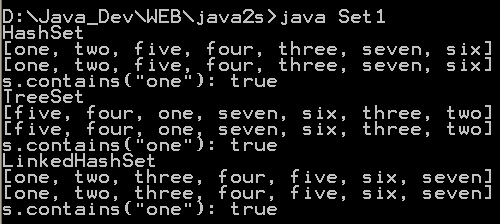3 Set operations: union, intersection, difference, symmetric difference, is subset, is superset 4 Set implementation that use == instead of equals() 5 Set that compares object by identity rather than equality 6 Set union and intersection 7 Set with values iterated in insertion order. 8 Putting your own type in a Set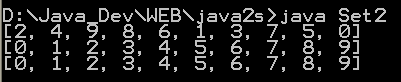9 Use set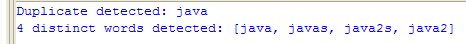10 Another Set demo 11 Set subtraction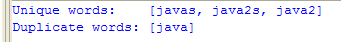12 Working with HashSet and TreeSet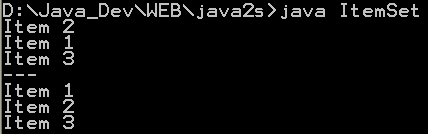13 TreeSet Demo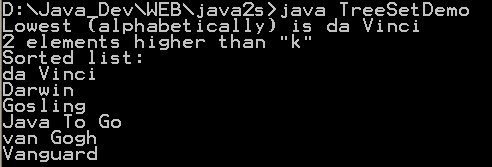14 Show the union and intersection of two sets 15 Demonstrate the Set interface 16 Array Set extends AbstractSet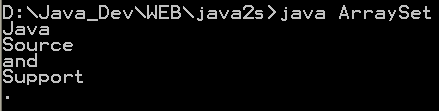17 Sync Test 18 Set Copy 19 Set and TreeSet 20 Tail 21 What you can do with a TreeSet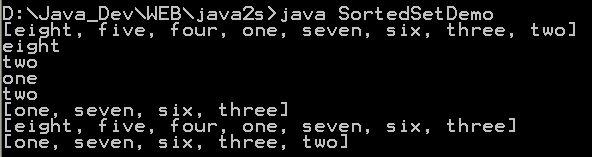22 Remove all elements from a set 23 Copy all the elements from set2 to set1 (set1 += set2), set1 becomes the union of set1 and set2 24 Remove all the elements in set1 from set2 (set1 -= set2), set1 becomes the asymmetric difference of set1 and set2 25 Get the intersection of set1 and set2, set1 becomes the intersection of set1 and set2 26 Extend AbstractSet to Create Simple Set 27 Int Set 28 One Item Set 29 Small sets whose elements are known to be unique by construction 30 List Set implements Set 31 Converts a char array to a Set 32 Converts a string to a Set 33 Implements the Set interface, backed by a ConcurrentHashMap instance 34 An IdentitySet that uses reference-equality instead of object-equality 35 An implementation of the java.util.Stack based on an ArrayList instead of a Vector, so it is not synchronized to protect against multi-threaded access. 36 A thin wrapper around a List transforming it into a modifiable Set. 37 A thread-safe Set that manages canonical objects 38 This program uses a set to print all unique words in System.in 39 Indexed Set 40 An ObjectToSet provides a java.util.Map from arbitrary objects to objects of class java.util.Set. 41 Sorted Multi Set 42 Fixed Size Sorted Set 43 Set operations 44 A NumberedSet is a generic container of Objects where each element is identified by an integer id. 45 Set which counts the number of times a values are added to it. 46 Set which counts the number of times a values are added to it and assigns them a unique positive index. 47 Indexed Set 48 A set acts like array. 49 Implements a Bloom filter. Which, as you may not know, is a space-efficient structure for storing a set. 50 Call it an unordered list or a multiset, this collection is defined by oxymorons